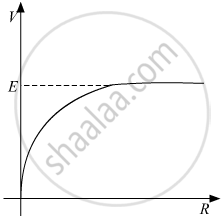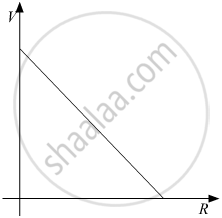# A cell of emf E and internal resistance r is connected across a variable resistor R. Plot the shape of graphs showing variation of terminal voltage V with (i) R and (ii) circuit current I. - Physics

Short Note

A cell of emf E and internal resistance r is connected across a variable resistor R. Plot the shape of graphs showing a variation of terminal voltage V with (i) R and (ii) circuit current I.

#### Solution

Terminal Voltage V can be related to R by the following relation:

(i) V = (ER)/(R+r)

⇒ V = (E)/(1+r/R)(ii) Terminal Voltage V can be related to I by the following relation:

V = E - IRConcept: Cells, Emf, Internal Resistance
Is there an error in this question or solution?